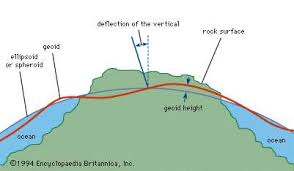## How to Calculate and Solve for Geoid Height Anomaly for an Ocean Basin | GravityThe image above represents genoid height anomaly for an ocean basin.

To compute for genoid height anomaly for an ocean basin, one essential parameter is needed and this parameter is depth (d).

The formula for calculating genoid height anomaly for an ocean basin:

Δh ≃ 3.85d (0.7 – 0.046d)

Where:

Δh = Geoid Height Anomaly for an Ocean Basin
d = Depth

Let’s solve an example;
Find the genoid height anomaly for an ocean basin when the depth is 20.

This implies that;

d = depth = 20

Δh ≃ 3.85d (0.7 – 0.046d)
Δh ≃ 3.85(20) (0.7 – 0.046(20))
Δh ≃ 77 (0.7 – 0.919)
Δh ≃ 77 (-0.219)
Δh ≃ -16.93

Therefore, the genoid height anomaly for an ocean basin is -16.93 m.